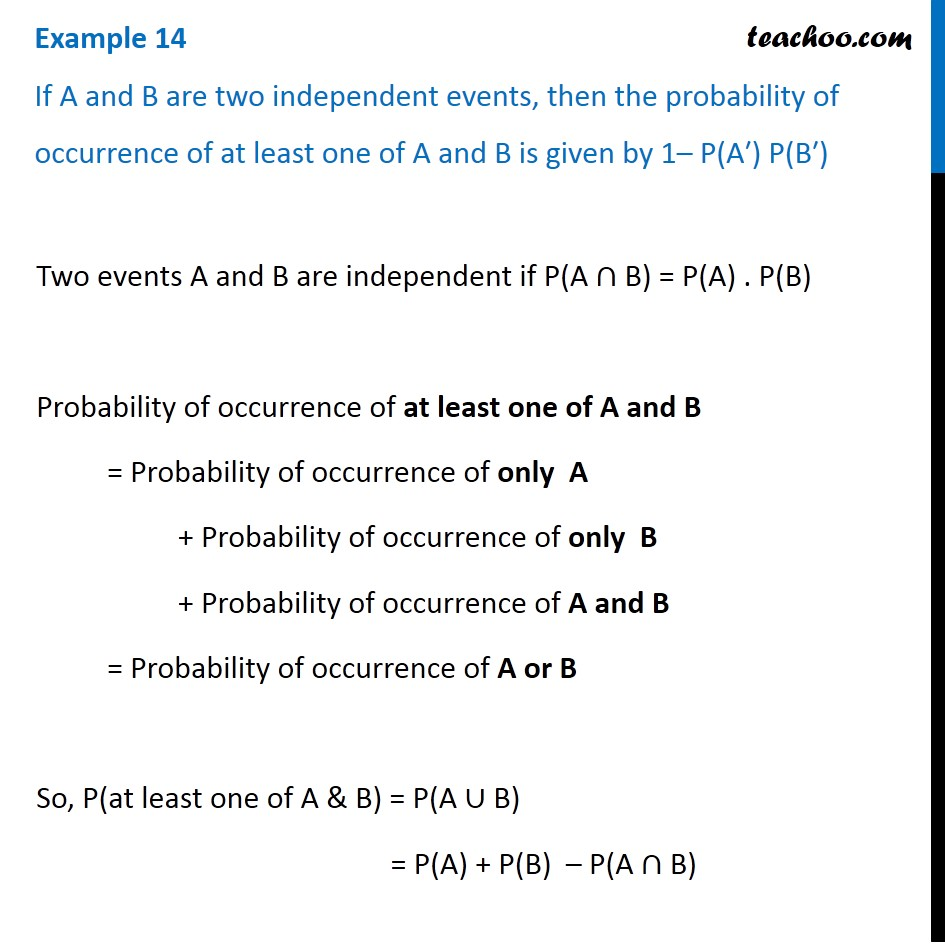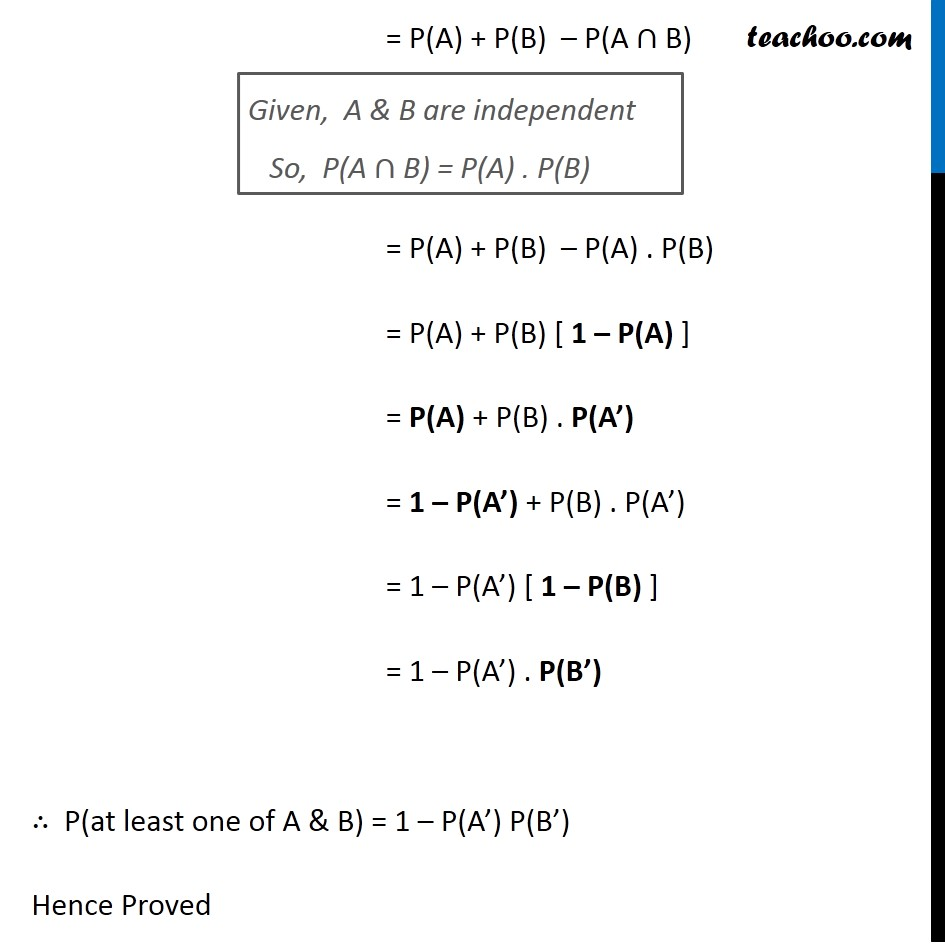Examples

Chapter 13 Class 12 Probability
Serial order wiseLearn in your speed, with individual attention - Teachoo Maths 1-on-1 Class

### Transcript

Example 14 If A and B are two independent events, then the probability of occurrence of at least one of A and B is given by 1– P(A′) P(B′) Two events A and B are independent if P(A ∩ B) = P(A) . P(B) Probability of occurrence of at least one of A and B = Probability of occurrence of only A + Probability of occurrence of only B + Probability of occurrence of A and B = Probability of occurrence of A or B So, P(at least one of A & B) = P(A ∪ B) = P(A) + P(B) – P(A ∩ B) = P(A) + P(B) – P(A ∩ B) = P(A) + P(B) – P(A) . P(B) = P(A) + P(B) [ 1 – P(A) ] = P(A) + P(B) . P(A’) = 1 – P(A’) + P(B) . P(A’) = 1 – P(A’) [ 1 – P(B) ] = 1 – P(A’) . P(B’) ∴ P(at least one of A & B) = 1 – P(A’) P(B’) Hence Proved Given, A & B are independent So, P(A ∩ B) = P(A) . P(B)